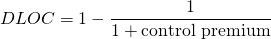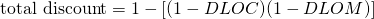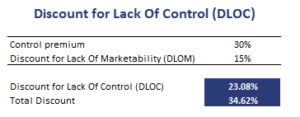# Discount for Lack of Control (DLOC)

The Discount for Lack Of Control (DLOC) is a discount that must be applied to the share price when the investor wishes to value a position in a company in which he or she will not have a controlling interest. In that case, the price obtained using, for example, a Free Cash Flow to the Firm (FCFF) valuation will be too high, because such a valuation assumes a controlling stake. A DLOC may sometimes be combined with a Discount for Lack of Marketability (DLOM).

On this page, we discuss the discount for lack of control definition, show how the DLOC can be derived from the control premium, and how to apply it to a share price. Finally, a spreadsheet at the bottom of the page illustrates all the formulas we discussed using a simple numerical example. The spreadsheet can be downloaded at the bottom of the page.

## Discount for lack of control definition

The DLOC is a discount that adjusts the price obtained using a valuation that assumes a control perspective. If we do not have a controlling interest, the price has to be reduced by a certain amount. The DLOC is mostly used when valuing private companies. The main determinants of the DLOC are transaction type, the industry conditions, and the type of consideration (stock versus cash).

## Discount for lack of control formula

Typically, the DLOC is derived from the control premium (CP). The reason why most analysts use a control premium is simply because it is difficult to measure the disadvantage from a lack of control. If we have the control premium, we can easily infer the DLOC as followsThe DLOC is strongly related to the discount for lack of marketability (DLOM). Often, the discount for lack of control and marketability are used together. This is the case when an analyst is valuing a minority stake investment in a private company. In that case, the total discount can be calculated as follows## Discount for lack of control example

To calculate control premiums, analysts typically use data from acquisitions of public companies. The following table illustrates how to calculate the discount for lack of control using the control premium. In addition to the DLOC, we also calculate the total discount.Once we have the DLOC, we can use it to adjust the value per share we obtained using a valuation approach such as a FCFE-based valuation.

## Summary

We discussed the DLOC, an important concept in the context of private equity valuation. The DLOC is typically derived from the control premium.

### How to calculate DLOC

Want to have an implementation in Excel? Download the Excel file: DLOC